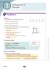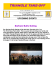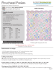# 5.1 Midsegment Theorem and Coordinate Proof

## Transcription

5.1 Midsegment Theorem and Coordinate Proof
```Geometry Chapter 5: Relationships within Triangles 5.1-­‐ Midsegment Theorem and Coordinate Proof SWBAT: use properties of midsegments and write coordinate proofs. Common Core: G.CO.10
Vocabulary A ______________________________ of a triangle is a segment that connects the midpoints of two sides of the triangle. Every triangle has three midsegments. Theorem 5.1-­‐ Midsegment Theorem The segment connecting the midpoints of two sides of a triangle is parallel to the third side and is half as long as that side. !
!!!!
𝐷𝐸 ∥ ________ and 𝐷𝐸 = ! ________
Example 1: Use the Midsegment Theorem 1. !!!!
𝐷𝐹 ∥ ________
2. !!!!
𝐸𝐹 ∥ ________
3. !!!!
𝐷𝐸 ∥ ________
4. 𝐴𝐵 = ________
5. 𝐷𝐸 = ________
6. 𝐴𝐶 = ________
Example 2: Use ∆𝐻𝐺𝐼 where 𝐿, 𝐾, 𝑎𝑛𝑑 𝐽 are midpoints 7. Find 𝐺𝐼.
8. Find 𝐾𝐽.
Geometry Chapter 5: Relationships within Triangles Use ∆𝐴𝐵𝐶 for problems 9 – 11, where D, E, and F are midpoints of the sides. 9. If 𝐷𝐸 = 2𝑥 + 6 𝑎𝑛𝑑 𝐶𝐵 = 6𝑥 + 4, 𝑤ℎ𝑎𝑡 𝑖𝑠 𝐷𝐸? 10. If 𝐷𝐹 = 3𝑥 + 3 𝑎𝑛𝑑 𝐴𝐵 = 8𝑥 − 4, 𝑤ℎ𝑎𝑡 𝑖𝑠 𝐴𝐸? 11. If 𝐴𝐶 = 4𝑥 − 2 𝑎𝑛𝑑 𝐸𝐹 = 𝑥 + 6, 𝑤ℎ𝑎𝑡 𝑖𝑠 𝐴𝐶? v A ___________________________________ proof involves placing geometric figures in a coordinate plane. 12. Graph ∆𝐴𝐵𝐶 with coordinates 𝐴 −1, 4 , 𝐵 3, 0 , 𝐶(5, 2). 𝑃 2, 3 is the midpoint of 𝐴𝐶. 𝑄(1, 2) is the midpoint of 𝐴𝐵. a) Prove that 𝑃𝑄 is parallel to 𝐵𝐶. (Use the slope formula.) b) Prove that 𝑃𝑄 is half as long as 𝐵𝐶. (Use the distance formula.) Geometry Chapter 5: Relationships within Triangles 13. Show that the midsegment 𝐹𝐺 is parallel to 𝐵𝐶 and half as long. ∆𝐴𝐵𝐶 has vertices 𝐴 −3, 4 , 𝐵 −3, −2 , 𝐶(5, 4). a) Find the coordinates of F, the midpoint of 𝐴𝐵. (Use the midpoint formula) b) Find the coordinates of G, the midpoint of 𝐴𝐶. (Use the midpoint formula) c) Verify that 𝐹𝐺 is parallel to 𝐵𝐶. (Use the slope formula to find the slopes of 𝐹𝐺 𝑎𝑛𝑑 𝐵𝐶) d) Verify that 𝐹𝐺 is half as long as 𝐵𝐶. (Use the distance formula to find the lengths of 𝐹𝐺 𝑎𝑛𝑑 𝐵𝐶) 5.1 Homework: Use the diagram of ∆𝐴𝐵𝐶 where 𝐷, 𝐸, 𝑎𝑛𝑑 𝐹 are the midpoints of the sides. 1. 𝐷𝐸 ∥ _________ 2. 𝐹𝐸 ∥ __________ 3. If 𝐴𝐵 = 26, then 𝐷𝐹 = __________ 4. If 𝐶𝐹 = 9, then 𝐷𝐸 = __________ 5. If 𝐸𝐹 = 7, then 𝐴𝐶 = __________ Geometry Chapter 5: Relationships within Triangles In the diagram of ∆𝐽𝐾𝑙 where 𝑅, 𝑆, 𝑎𝑛𝑑 𝑇 are the midpoints of the sides and 𝑅𝐾 = 6, 𝐾𝑆 = 8, 𝑎𝑛𝑑 𝐽𝐾 ⊥ 𝐾𝐿. 6. Find the length of 𝑅𝑆. (Pythagorean Theorem) 7. Find the length of 𝐽𝐾. 8. Find the length of 𝑅𝑇. 9. Find the perimeter of ∆𝐽𝐾𝐿. 10. Name all of the right angles in the diagram. Use the diagram of ∆𝑀𝑁𝑂 where 𝑋, 𝑌, 𝑎𝑛𝑑 𝑍 are the midpoints of the sides. 11. If 𝑌𝑍 = 3𝑥 + 1 and 𝑀𝑁 = 10𝑥 − 6 then 𝑌𝑍 = __________. 12. If 𝑌𝑋 = 𝑥 − 1, and 𝑀𝑂 = 3𝑥 − 7, then 𝑀𝑂 = __________. 13. If 𝑚∠𝑀𝑂𝑁 = 48°, then 𝑚∠𝑀𝑍𝑋 = __________. 14. If 𝑚∠𝑀𝑋𝑍 = 37°, then 𝑚∠𝑀𝑁𝑂 = __________. 15. Name a triangle that appears to be congruent to ∆𝑍𝑂𝑌. ```

### The Midpoint Formula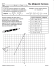### Algebra with shapes How to . . .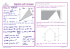### Coordinate Geometry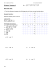### Solve the problems below to answer the riddle: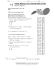### HSM12CC_GM_06_09_CM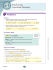### Lesson 30: Special Lines in Triangles M1 Lesson 30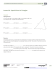### Reteach Sec 1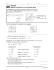### Coordinate Geometry Unit Test Review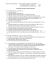### See details. - Math Awareness Month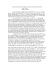### 1-2 Practice A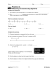### HSM12CC_GM_06_07_CM - Newark Catholic High School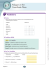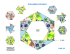### wizBRAIN - W4Kangoeroe### Midsegments of Triangles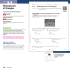### 10.3 Coordinate Proof Using Distance with Segments and Triangles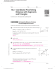### The “Elusive Formulas” Section A### Lesson 12: Dividing Segments Proportionately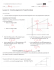### Scrappy Star Quilt - Art Gallery Fabrics### VA517571_GM Release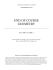### Midsegments of Triangles